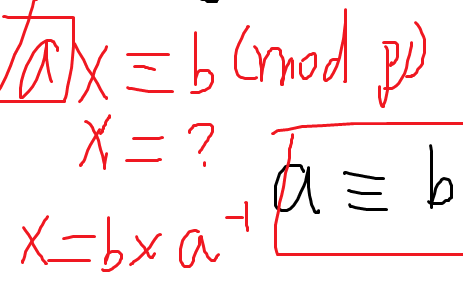## qbzt day2 晚上（竟然还有晚上）

A*

g 当前点到根节点的深度

h 当前点到终点理想的最优情况需要走几步

f  f=g+h

A*就是把所有的f从小到大排序

g*(x) : S x 的理论最近距离

g(x) : S x 对于 g*(x) 的估计

f*(x) : x T 的理论最近距离,F*(x)=g*(x)+h*(x)

f(x) : x T 对于 f*(x) 的估计,F(x)=g(x)+h(x)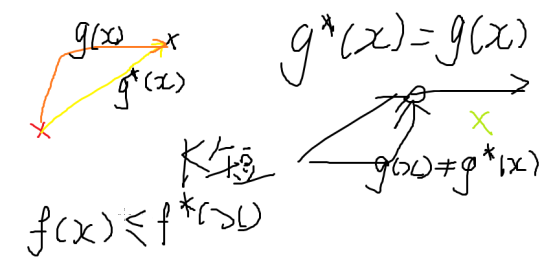BFS 几乎一样，只是每次都弹出当前局面中f(x)最小的那个局面进行扩展

——故需要维护一个优先队列（小根堆）

——使用系统的priority_queue<>即可

f(x) = g(x) + h(x) h(x) = 0 即失去了启发式函数，则变为Breath First Search

f(x) = g(x) + h(x) g(x) = 0 则变为 Best First Search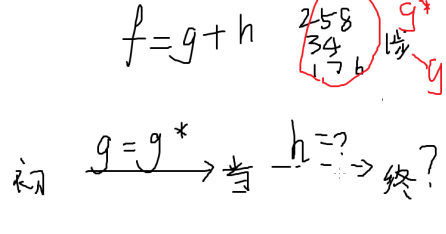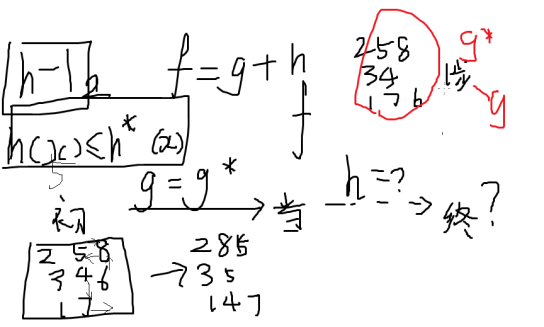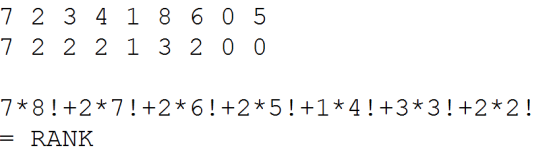IDA*

g：从根节点往下几步

h：步数

g+h>d return

h(x)>h*(x)?

h(x)越接近h*(x)跑得越快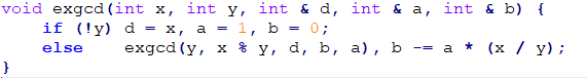x 对一个数 p 有逆元当且仅当 (x, p) = 1

……，等等，岂不是 ax == 1 (mod p)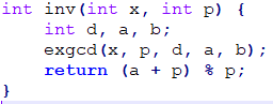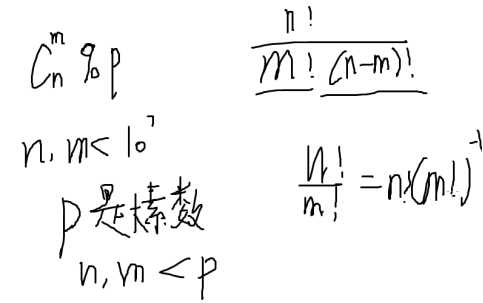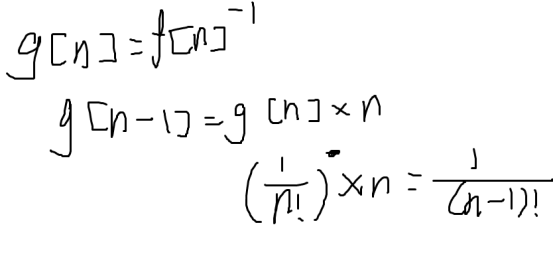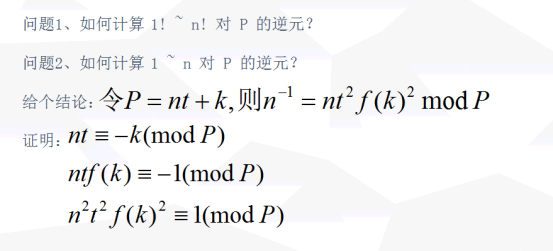P = p1 * p2 * ... * pn

Pi = P / pi

Qi = Pi pi 的逆元

x = sigma(ai * Pi * Qi)